﻿ A Class of Single-CFOA-based Sinusoidal OscillatorsPublications are Open
Access in this journal
Article Versions
Export Article
• Normal Style
• MLA Style
• APA Style
• Chicago Style
Original Article
Open Access Peer-reviewed

A Class of Single-CFOA-based Sinusoidal Oscillators

Dharmesh Kumar Srivastava, Vinod Kumar Singh, Raj Senani
American Journal of Electrical and Electronic Engineering. 2020, 8(1), 1-10. DOI: 10.12691/ajeee-8-1-1
Received November 03, 2019; Revised December 24, 2019; Accepted December 29, 2019

Abstract

A class of second order sinusoidal oscillators is presented which employs a single Current feedback-operational amplifier, four capacitors and four to five resistors which can be realized by CMOS linear voltage controlled resistors (VCR). The proposed circuits are simultaneously single resistance controlled oscillators (SRCO) as well as single capacitor controlled oscillators (SCCO) providing independent control of condition of oscillation and frequency of oscillation through independent resistors as well as independent capacitors. To the best knowledge of the authors, any single-CFOA-based oscillators which are simultaneously SRCOs as well as SCCOs have not been reported in the literature earlier. SPICE simulation results using 0.18μm CMOS technology have been given which confirm the validity of the proposed circuits.

1. Introduction

Because of the significant advantages offered by Current feedback operational amplifiers (CFOA) in realizing sinusoidal oscillators (see 1, 2) there have been a number of studies on oscillator realization using CFOAs for instance, see 3, 4, 5, 6, 7, 8, 9, 10, 11, 12, 13, 14, 15, 16, 17, 18, 19, 20, 21, 22, 23 and the references cited in 24, 25 and 26. However a lot of work on oscillators is done employing other analog building blocks also for instance, see 27, 28, 29, 30, 31 and 32, 33, 34, 35, 36, 37, 38, 39, 40, 41, 42, but no such block has yet attained the same status as CFOA because CFOAs are available commercially as off the shelf ICs out of which AD-844 has been widely used to practically implement CFOA-based oscillators.

A majority of the earlier works, however, have prominently focused on the realization of the so-called canonic single resistance controlled oscillators (SRCO) i.e. those oscillators in which condition of oscillation (CO) and frequency of oscillation (FO) can be controlled/ adjusted by means of single variable resistors. Such oscillators typically require, besides a single CFOA, three resistors and two capacitors. On the other hand, the single capacitor controlled oscillators (SCCO) have been considered very rarely; one isolated example being that of 24.

In fact, during most of the earlier investigations 1, 2, 3, 4, 5, 6, 7, 8, 9, 10, 11, 12, 13, 14, 15, 16, 17, 18, 19, 20, 21, 22, 23 the non-canonic oscillators have, by and large, been neglected on the presumption that they may not have any interesting features to offer. By contrast, recently, the authors have demonstrated in 43 and 44 that some non-canonic realizations of CFOA-based oscillators may, indeed, possess some interesting and practically important properties which may not have been realized in the canonic oscillators. In particular, in 43 it was shown that by permitting the use of more than the canonic number of capacitors, it becomes possible to derive sinusoidal oscillators suitable for the generation of very low frequency (VLF) oscillations thus, exhibiting a feature which does not exist in any of the previously known VLF oscillators made from a single CFOA. On the other hand, in 44, it has been shown that a specific single-CFOA-based non-canonic oscillator employing three capacitors makes it possible to absorb all the parasitic impedances of the CFOA terminals at x, y and z into the various external components employed, thereby significantly reducing the error between the practically observed frequency values and those predicted from the non-ideal formulae.

Encouraged by these developments 43, 44 therefore, in this paper, we focus again on non-canonic single-CFOA-based oscillators and present a number of new configurations to give further evidence that, indeed, some non-canonic structures can really possess properties which may not be available in the canonic oscillators. In particular, we introduce two families of single-CFOA-based oscillators, both of which are non-canonic with respect to the number of resistors and capacitors used but have the novel feature that they are simultaneously SRCOs as well as SCCOs with completely uncoupled tuning laws. To the best knowledge of the authors, any single-CFOA-based oscillator configurations exhibiting these properties do not appear to have been reported in the open literature earlier.

The workability of the new circuits has been substantiated by SPICE simulation results. Furthermore, the SPICE simulation results of their completely CMOS-compatible versions realizing linear voltage-controlled-oscillators (VCO) have also been appended.

2. The Proposed Configurations

Before presenting the new class of circuits, we may recall that a CFOA is a four terminal active building block represented schematically as shown in Figure 1, with its terminal equations as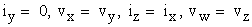(1)
• Figure 1. Symbolic notation and the equivalent circuit of the CFOA

In the following, a catalogue of eight single-CFOA-based non-canonic SRCOs is derived which can be used as SRCOs as well as SCCOs. The proposed configurations are derived from the general five node structure using a single CFOA shown in Figure 2.

• Figure 2. General five-node structure

By a standard analysis, the characteristic equation of this circuit is found to be:(2)

From the above structure and the generalized characteristic equation (2), all possible circuits of SRCOs and SCCOs which belong to the intended type were searched. This search has culminated into eight possible sinusoidal oscillator configurations of the desired type, resulting from the generalized structure of Figure 2, which are shown in Figure 3.

The expressions for the CO and the FO of these circuits have been summarized in Table 1.

• Figure 3. The derived SRCO/SCCO structures

3. Effect of the CFOA Parasitics

It is useful to examine the effect of the various non-ideal parameters of the CFOAs on the frequency of oscillation of the various circuits. Taking into consideration the finite x-terminal input resistance Rx, finite y-terminal parasitic impedances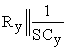and z-terminal parasitic impedance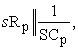these have been derived and are shown in Table 1. However, from an inspection of Figure 3and Table 1, it may be seen that the oscillator circuits A, B, C and D are suitable to be operated as VLF oscillators (in sub-audio range i.e. <20Hz). In such frequency ranges, the effect of parasitic impedances of the CFOA is not likely to have any significant effect and hence, such an analysis is not required to be carried out for these circuits. On the other hand, circuits E, F, G and H of Figure 3 appear to be suitable for generating medium to high frequency oscillations and therefore, only the results of the non-ideal analysis of these circuits are shown in Table 2. Since the expressions are quite complex it is difficult to make out any qualitative interpretations from these, therefore, the deviations in the oscillation frequencies realized due to effect of various parasitics have been studied through SPICE simulations and MATLAB-based plots using these expressions and have been presented subsequently.

4. Sensitivity and Frequency Stability

The various sensitivity figures for the various oscillators have been given in Table 3. Besides this, the frequency stability factor is another important figure of merit which has also been evaluated. The frequency stability factor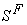is defined as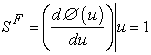whereand Φ(u) is the phase function of the open loop transfer function of the oscillator circuit. The frequency stability factors for the various circuits, so determined, are also given in Table 3.

From an inspection of Table 3, it may be seen that since all the three-capacitor oscillators (circuits A, B, C and D) are essentially VLF oscillators, there is a difference term in the numerator of the expression for the oscillation frequency as a consequence of which these circuits are bound to have some sensitivity figures (particularly those with respect to the frequency controlling parameter) much larger than others. Furthermore, because of the same reason, these circuits would also have smaller values of the frequency stability factor. On the other hand, the four capacitor oscillators (circuits E, F, G and H) of Table 3 enjoy low sensitivity properties and can have moderate to high values of stability factors when the parameter n is varied over a judiciously chosen range of values.

By varying the value of n from 0.1 to 0.99 for circuits A, B, C and D, the frequency stability factor varies from -1.276 to 1.45 (for n=0.1) and -0.12 to -0.125 9 (for n=0.99). Similarly, varying the value of n from 1,10 and 100 for circuits E, F, G and H, the frequency stability factors are found to be -3.03, 0.19, -0.25 and -1.732 (for n=1), -8.70, -1.6, -0.5 and 1.04 (for n=10) and -28.15,-8.82, -0.365 and 6.66 (for n=100) respectively.

5. SPICE Simulation Results

The workability of all the derived oscillator circuits has been verified by SPICE simulations using AD844 macro-model for the CFOA as well as using CMOS CFOAs along with CMOS VCRs. All the circuits have been found to work almost as predicted by theory. Here, we present some sample simulation results.

• Figure 4. SPICE simulation results of Circuit-B of Figure 3 ((a) A typical waveform obtained from the circuit-B in SRCO-mode (13.76Hz, 7.27Volts p-p) for component values C2=C3=100nFand C4=200nF, R0= R1=100KΩ, R4=350KΩ. (b) A typical waveform obtained from the circuit-B for SCCO-mode (983.33mHz, 7.2V p-p) for component values C2=250nF, C3=100nF and C4=150nF-322nF, R4=600KΩ R0=R1=100KΩ).
• Figure 5. SPICE simulation results for Circuit B (a) variation of frequency with R4 (b)variation of frequency with C2

The oscillator Circuit-B was simulated by taking supply voltage of the AD844 type CFOA as ±12V and taking R0=R1=100KΩ, R4=350KΩ, R2=104KΩ and C2=C3= 100 nF, C4=200nF. The oscillation frequency obtained from simulations was found to be 13.76Hz against a theoretical value of 13.43Hz for SRCO mode of circuit-B. On the other hand, with R0=R1=500KΩ, R4=600KΩ, C3=100nF, C2=250nF and C4=150nF-322nF, the oscillation frequency obtained was 983.33 mHz against the theoretical value of 821.06mHzfor the SCCO mode of circuit-B. The simulation results and the variation of frequency with respect to resistance R4 and capacitanceC2 for circuit-B have been shown in Figure 4(a), (b) and Figure 5 respectively.

Similarly, the oscillator Circuit-G was simulated by taking supply voltages of the AD844 CFOA as ±12V, R0=R3=150KΩ, R7=5KΩ with variable R4=46KΩ (40KΩ fixed+10KΩ variable), C0=50pF, C4=10pF, C6=100pF and C7=25pF.The oscillation frequency obtained from simulations was 121 KHz against the intended value of 120.99 KHz for the SRCO mode of circuit-G. On the other hand, with R0=R3=150KΩ, R7=10KΩ, R4=50KΩ and C0=100pF, C4=70pF, C6=150pF and C7=15pF, the oscillation frequency obtained from the simulations was found to be 80.15 KHz against the intended value of 87.63 KHz for SCCO mode of circuit-G. The simulation results and the variation of frequency with respect to resistance R7and capacitance C4 for circuit-G have been shown in Figure 6(a), (b) and Figure 7 respectively, which confirm the workability of this circuit.

• Figure 6 SPICE simulation results of the Oscillator-G of Figure 3 ((a) A typical waveform (121 KHz, 7.2Volts p-p)for component values C0=50pF, C6=100pF,C4=10 pf,C7=25pF and R7=5KΩ, R0= R3=150KΩ, R4=46KΩ. (b) A typical waveform (80.15 KHz, 7.4Volts p-p) for component values C0=100pF, C6=150pF, C4=70pF, C7=15pFandR0= R3=150KΩ, R4=50K Ω, R7=10KΩ).
• Figure 7. SPICE simulation results for the oscillator circuit-G (a) variation of oscillation frequency with R7 (b)variation of oscillation frequency with C4

6. Realization of Linear Voltage-Controlled Oscillators and Their Simulation Results

For realizing linear VCOs implementable in CMOS Technology, the CFOA needs to be realized in CMOS for which a number of alternatives have been proposed in recent literature. For the present work, we have chosen the CMOS CFOA of Chen and Wu 45 which is reproduced here in Figure 8. All the resistors contained in the circuits of Figure 3, whether floating or grounded, were considered to be implemented in CMOS through the common CMOS linear floating VCR circuit from 46, 47 which is also reproduced here in Figure 9 for a ready reference.

To illustrate how the oscillator circuits E, F, G and H of Figure 3 can be converted into linear CMOS VCOs, we consider the circuit E of Figure 3. In this circuit if R0, R3, R4 and R7 are replaced by a CMOS VCRs, in which R0 and R3 are derived by a common control voltage VC03, whereas R4 and R7are derived by different control voltages VC04 and VC07. Now, noting that the equivalent resistance simulated by the circuit of 46, 47 is given by(3)

where, K0 and Kt are transistor’s trans-conductance and threshold voltage respectively, the expression for FO would therefore is modified as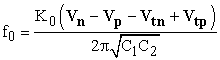(4)

Therefore, it is clear that oscillation frequency would be a linear function of the common control voltage Vn because Vp is fixed at 0.9V and the circuit would, thus, realize a linear VCO.

For SPICE simulations, the W/L ratios of the MOSFETs used for implementing the CFOA (Figure 8) were taken to be as given in Table 4 and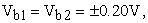whereas the aspect ratios of the MOSFETs used to realize the floating and grounded VCR circuit of Figure 9 were taken for PMOS and NMOS as (W/L=2.5µm/0.18µm) and (W/L=0.15µm/0.18µm) with the model parameters of 0.18µm CMOS Technology adopted from TSMC as shown in Table 5.

• Figure 9. CMOS floating voltage controlled resistor [26,46]
• Figure 10. SPICE simulation result of the circuit E in linear VCO mode: Frequency (as obtained from simulation) is 10.00MHz, THD=1.68% with component values C0=5pF, C1=50pF, C7=10pF, C4=10pF, VC0=VC3=0.4V, Vc4=1.1V and Vc7=0.5V and Vp=-0.9V.

The SPICE simulation results for circuit-E of Figure 3 as the linear VCOs are given in Figure 10 and Figure 11 which show a typical waveform and the variation of oscillation frequency with control voltage.

These SPICE simulation results, which show a good compliance, thus, establish the realisability of the linear VCO.

• Figure 11. Variation of oscillation frequency with the control voltage for oscillator Circuit-E

7. Comparison between Different Proposed Oscillator Circuits

From the calculations based upon the non-ideal expressions for oscillation frequencies (omitted here to conserve space) it has been found that oscillator circuit-A of Figure 3 has the smallest deviation between the theoretical and SPICE-generated values of oscillation frequency. Furthermore, although it has been possible to generate low frequencies with all of them but circuit-B appears to be the best in this respect since in the SCCO-mode, this circuit enables the generation of oscillation frequency as low as about 1Hz.

Similarly, all the four-capacitors-based SRCOs/SCCOs have been tested through SPICE simulations and the variation of frequency with respect to the frequency controlling variable resistor as well as frequency-controlling variable capacitor have been observed and have been found to be as predicted by the theory, as shown in Figure 6 and Figure 7.

In addition, we have also verified the workability of the SRCO-mode by converting the circuits into VCOs by replacing all the resistors by a two MOSFET based floating VCR 46, 47 and using CMOS CFOA of Chen and Wu 45. A typical output waveform obtained from SPICE simulations and the variations of the oscillation frequency with control voltage are shown in Figure 10 and Figure 11. It has been found that all the four circuits can be successfully used as linear VCOs as envisaged.

8. Concluding Remarks

Whereas a large number of papers have been published on the realization of canonic sinusoidal oscillators using a single CFOA, the non-canonic structures have been largely neglected in the earlier literature on the presumption that they may not have any interesting features. In this paper, we have presented a class of new non-canonic single-CFOA-based oscillators and show that contrary to the popular belief, the presented non-canonic oscillators do possess an interesting feature in that they are simultaneously SRCOs as well as SCCOs providing independent control of condition of oscillation and frequency of oscillation in both the modes through separate circuit elements. The workability of some of the new oscillator circuits has been demonstrated by SPICE simulations using AD844 macro-model. Completely CMOS versions of the various circuits operated as linear VCOs have also been verified through SPICE simulations by replacing the passive resistors by linearized CMOS linear voltage controlled resistors and using CMOS CFOAs and some sample results have been presented. All the simulation results have confirmed the workability of the proposed formulations. In view of this, it is believed that this paper has added new kind of circuits to the existing repertoire of single-CFOA-based sinusoidal oscillators 1, 2, 3, 4, 5, 6, 7, 8, 9, 10, 11, 12, 13, 14, 15, 16, 17, 18, 19, 20, 21, 22, 23.

References

Published with license by Science and Education Publishing, Copyright © 2020 Dharmesh Kumar Srivastava, Vinod Kumar Singh and Raj SenaniThis work is licensed under a Creative Commons Attribution 4.0 International License. To view a copy of this license, visit http://creativecommons.org/licenses/by/4.0/